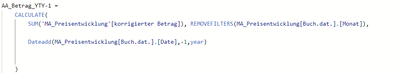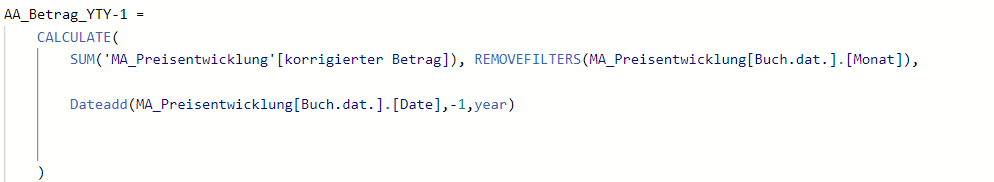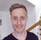cancel
Showing results for
Did you mean:Anonymous
Not applicable

## sum complete last year (Year to Year -1)

Hey Guys,

I want to measure the Total amount of the last year (YTY-1).

But i have one restiiction: I am using a measure of the YTD. If I filter the month, I get the YTY-1 with filtered values too. But it should measure the total value of the last year

Best regards from Germany 🙂

my current measure:1 ACCEPTED SOLUTIONSolution Sage

Hi!

Do you have a Date table (Set and use date tables in Power BI Desktop - Power BI | Microsoft Learn)?
If so, PREVIOUSYEAR() should work, in the form of:

``````AA_Betrag_YTY-1 :=
CALCULATE(
[korrigierter Betrag], //assuming this is the measure you want to calculate
PREVIOUSYEAR(Dates[Date]) //date column from your date table
)``````

2 REPLIES 2Solution Sage

Hi!

Do you have a Date table (Set and use date tables in Power BI Desktop - Power BI | Microsoft Learn)?
If so, PREVIOUSYEAR() should work, in the form of:

``````AA_Betrag_YTY-1 :=
CALCULATE(
[korrigierter Betrag], //assuming this is the measure you want to calculate
PREVIOUSYEAR(Dates[Date]) //date column from your date table
)``````Anonymous
Not applicable

Hi Tomas

yeah, it works well. I know one more function now too. Thanks for that!